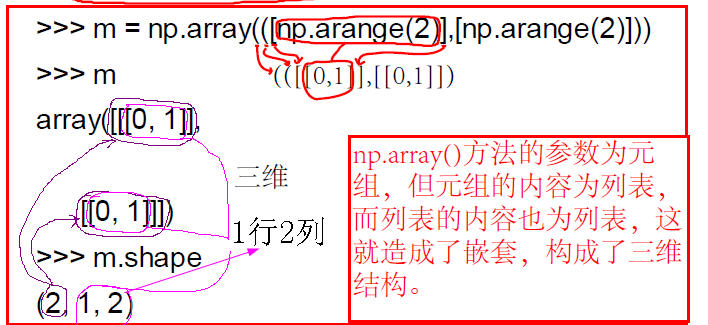# 开篇

numpy库作为科学计算的基础库，其地位相当重要，它是对数组操作的基石。它的存在使得线性代数以及矩阵论等相关知识在计算机上的表达更加方便与简单，集中体现出了人想办法，计算机去工作。

Python的numpy库的安装百度一下即可解决，部分Python3已将numpy库作为内置库，可以不安装。

# 1导入模块库numpy

import numpy as np

# 2一维数组的简单创建# 3二维数组的简单创建## 1.Numpy（Numerical Python）

Numpy：提供了一个在Python中做科学计算的基础库，重在数值计算，主要用于多维数组（矩阵）处理的库。用来存储和处理大型矩阵，比Python自身的嵌套列表结构要高效的多。本身是由C语言开发，是个很基础的扩展，Python其余的科学计算扩展大部分都是以此为基础。

• 高性能科学计算和数据分析的基础包

• ndarray，多维数组（矩阵），具有矢量运算能力，快速、节省空间

• 矩阵运算，无需循环，可完成类似Matlab中的矢量运算

• 线性代数、随机数生成

• `import numpy as np`

## 2.ndarray 多维数组(N Dimension Array)

NumPy数组是一个多维的数组对象（矩阵），称为ndarray，具有矢量算术运算能力和复杂的广播能力，并具有执行速度快和节省空间的特点。

# 3三维数组的创建-采用嵌套方案### ndarray拥有的属性

`ndim属性：`维度个数
`shape属性：`维度大小
`dtype属性：`数据类型

## ndarray的随机创建

``````# 导入numpy，别名np
import numpy as np

# 生成指定维度大小（3行4列）的随机多维浮点型数据（二维），rand固定区间0.0 ~ 1.0
arr = np.random.rand(3, 4)
print(arr)
print(type(arr))

# 生成指定维度大小（3行4列）的随机多维整型数据（二维），randint()可以指定区间（-1, 5）
arr = np.random.randint(-1, 5, size = (3, 4)) # 'size='可省略
print(arr)
print(type(arr))

# 生成指定维度大小（3行4列）的随机多维浮点型数据（二维），uniform()可以指定区间（-1, 5）
arr = np.random.uniform(-1, 5, size = (3, 4)) # 'size='可省略
print(arr)
print(type(arr))

print('维度个数: ', arr.ndim)
print('维度大小: ', arr.shape)
print('数据类型: ', arr.dtype)
``````

``````[[ 0.09371338  0.06273976  0.22748452  0.49557778]
[ 0.30840042  0.35659161  0.54995724  0.018144  ]
[ 0.94551493  0.70916088  0.58877255  0.90435672]]
<class 'numpy.ndarray'>

[[ 1  3  0  1]
[ 1  4  4  3]
[ 2  0 -1 -1]]
<class 'numpy.ndarray'>

[[ 2.25275308  1.67484038 -0.03161878 -0.44635706]
[ 1.35459097  1.66294159  2.47419548 -0.51144655]
[ 1.43987571  4.71505054  4.33634358  2.48202309]]
<class 'numpy.ndarray'>

``````

# 4代码验证吧

``````>>> import numpy as np
>>> a = np.arange(10)
>>> a
array([0, 1, 2, 3, 4, 5, 6, 7, 8, 9])
>>> a = np.arange(1,10)
>>> a
array([1, 2, 3, 4, 5, 6, 7, 8, 9])
>>> a = np.arange(1,10,3)
>>> a
array([1, 4, 7])
>>> #2D
>>> M = np.array([[1,2],[3,4]]) #参数为列表
>>> M
array([[1, 2],
[3, 4]])
>>> M = np.array(([1,2],[3,4])) #参数为元组
>>> M
array([[1, 2],
[3, 4]])
>>> M = np.array(((1,2),(3,4))) #参数为元组
>>> M
array([[1, 2],
[3, 4]])

>>> M = np.array(((np.arange(3)), (np.arange(3))))
>>> M
array([[0, 1, 2],
[0, 1, 2]])
>>> M.shape
(2, 3)
>>> M = np.array(([np.arange(2)],[np.arange(3)]))
>>> M
array([[array([0, 1])],
[array([0, 1, 2])]], dtype=object)
>>> M.shape
(2, 1)
>>> #3D
>>> M = np.array(([np.arange(2)],[np.arange(2)]))
>>> M
array([[[0, 1]],

[[0, 1]]])
>>> M.shape
(2, 1, 2)
``````

## 3.ndarray的序列创建

### 1. np.array(collection)

collection 为 序列型对象(list)、嵌套序列对象(list of list)。

``````# list序列转换为 ndarray
lis = range(10)
arr = np.array(lis)

print(arr)            # ndarray数据
print(arr.ndim)        # 维度个数
print(arr.shape)    # 维度大小

# list of list嵌套序列转换为ndarray
lis_lis = [range(10), range(10)]
arr = np.array(lis_lis)

print(arr)            # ndarray数据
print(arr.ndim)        # 维度个数
print(arr.shape)    # 维度大小
``````

``````# list序列转换为 ndarray
[0 1 2 3 4 5 6 7 8 9]
1
(10,)

# list of list嵌套序列转换为 ndarray
[[0 1 2 3 4 5 6 7 8 9]
[0 1 2 3 4 5 6 7 8 9]]
2
(2, 10)
``````

### 4. np.empty()

``````# np.zeros
zeros_arr = np.zeros((3, 4))

# np.ones
ones_arr = np.ones((2, 3))

# np.empty
empty_arr = np.empty((3, 3))

# np.empty 指定数据类型
empty_int_arr = np.empty((3, 3), int)

print('------zeros_arr-------')
print(zeros_arr)

print('n------ones_arr-------')
print(ones_arr)

print('n------empty_arr-------')
print(empty_arr)

print('n------empty_int_arr-------')
print(empty_int_arr)
``````

``````------zeros_arr-------
[[ 0.  0.  0.  0.]
[ 0.  0.  0.  0.]
[ 0.  0.  0.  0.]]

------ones_arr-------
[[ 1.  1.  1.]
[ 1.  1.  1.]]

------empty_arr-------
[[ 0.  0.  0.]
[ 0.  0.  0.]
[ 0.  0.  0.]]

------empty_int_arr-------
[[0 0 0]
[0 0 0]
[0 0 0]]
``````

### 5. np.arange() 和 reshape()

arange() 类似 python 的 range() ，创建一个一维 ndarray 数组。

reshape() 将 重新调整数组的维数。

``````# np.arange()
arr = np.arange(15) # 15个元素的 一维数组
print(arr)
print(arr.reshape(3, 5)) # 3x5个元素的 二维数组
print(arr.reshape(1, 3, 5)) # 1x3x5个元素的 三维数组
``````

``````[ 0  1  2  3  4  5  6  7  8  9 10 11 12 13 14]

[[ 0  1  2  3  4]
[ 5  6  7  8  9]
[10 11 12 13 14]]

[[[ 0  1  2  3  4]
[ 5  6  7  8  9]
[10 11 12 13 14]]]
``````

### 6. np.arange() 和 random.shuffle()

random.shuffle() 将打乱数组序列（类似于洗牌）。

``````arr = np.arange(15)
print(arr)

np.random.shuffle(arr)
print(arr)
print(arr.reshape(3,5))
``````

``````[ 0  1  2  3  4  5  6  7  8  9 10 11 12 13 14]

[ 5  8  1  7  4  0 12  9 11  2 13 14 10  3  6]

[[ 5  8  1  7  4]
[ 0 12  9 11  2]
[13 14 10  3  6]]
``````